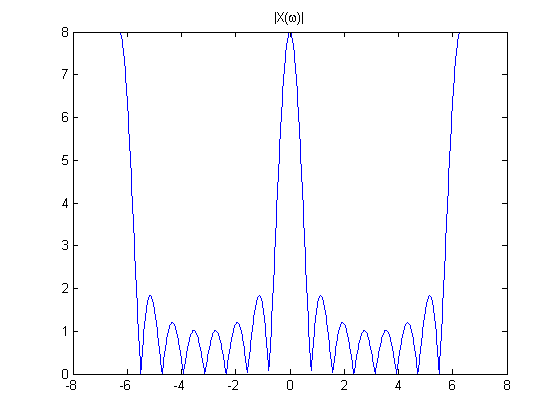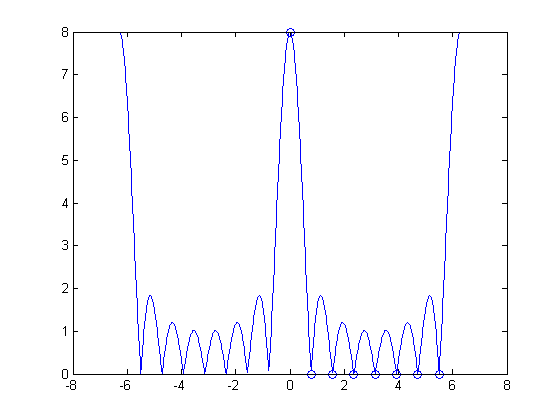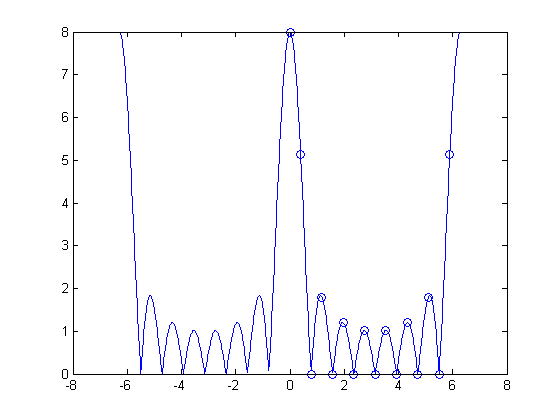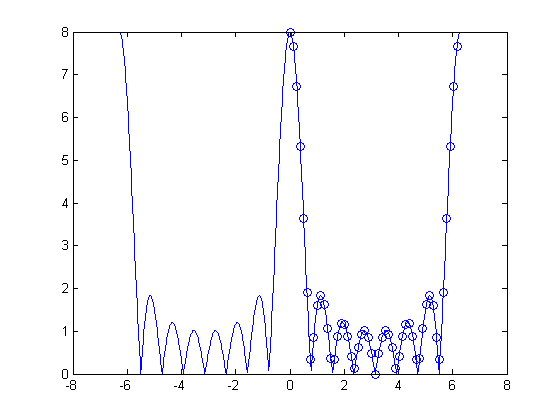# The DFT and the DTFT

It's finally time to start looking at the relationship between the discrete Fourier transform (DFT) and the discrete-time Fourier transform (DTFT). Let's look at a simple rectangular pulse, $x[n] = 1$ for $0 \leq n < M$. The DTFT of $x[n]$ is:

Let's plot $|X(\omega)|$ for $M=8$ over a couple of periods:

M = 8;
w = linspace(-2*pi, 2*pi, 800);
X_dtft = (sin(w*M/2) ./ sin(w/2)) .* exp(-1j * w * (M-1) / 2);
plot(w, abs(X_dtft))
title('|X(\omega)|')It turns out that, under certain conditions, the DFT is just equally-spaced samples of the DTFT. Suppose $X_P[k]$ is the P-point DFT of $x[n]$. If $x[n]$ is nonzero only over the finite domain $0 \leq n < M$, then $X_P[k]$ equals $X(\omega)$ at equally spaced intervals of $\omega$:

The MATLAB function fft computes the DFT. Here's the 8-point DFT of our 8-point rectangular pulse:

x = ones(1, M);
X = fft(x)

X =

8     0     0     0     0     0     0     0



One 8 and a bunch of zeros?? That doesn't seem anything like the DTFT plot above. But when you superimpose the output of fft in the right places on the DTFT plot, it all becomes clear.

P = 8;
w_k = (0:P-1) * (2*pi/P);
X = fft(x);
plot(w, abs(X_dtft))
hold on
plot(w_k, abs(X), 'o')
hold offNow you can see that the seven zeros in the output of fft correspond to the seven places (in each period) where the DTFT equals zero.

You can get more samples of the DTFT simply by increasing P. One way to do that is to zero-pad.

x16 = [x, zeros(1, 8)]

x16 =

Columns 1 through 13

1     1     1     1     1     1     1     1     0     0     0     0     0

Columns 14 through 16

0     0     0


P = 16;
X16 = fft(x16);
w_k = (0:P-1) * (2*pi/P);
X = fft(x);
plot(w, abs(X_dtft))
hold on
plot(w_k, abs(X16), 'o')
hold offAnother way to increase P is to use the fft(x,P) syntax of the fft function. This syntax computes the P-point DFT of x by using zero-padding. Let's try a 50-point DFT.

P = 50;
Xp = fft(x, P);
w_k = (0:P-1) * (2*pi/P);
X = fft(x);
plot(w, abs(X_dtft))
hold on
plot(w_k, abs(Xp), 'o')
hold offIf you've ever wondered what that whole zero-padding business was all about with Fourier transforms, now you know. When you tack on a bunch of zeros to a sequence and then compute the DFT, you're just getting more and more samples of the DTFT of the original sequence.

I think the next logical place to go in our Fourier exploration is to start considering some of the reasons why many people find the output of fft so surprising or puzzling. Here's a sample:

• Why isn't the zero frequency (or "DC" frequency) in the center of the output from fft?
• Why isn't the output of fft real when the input is symmetric?